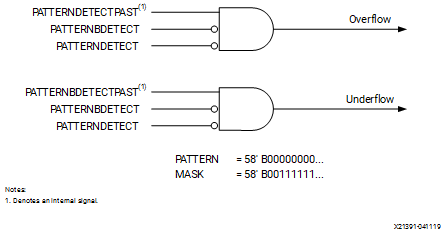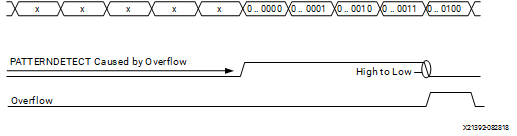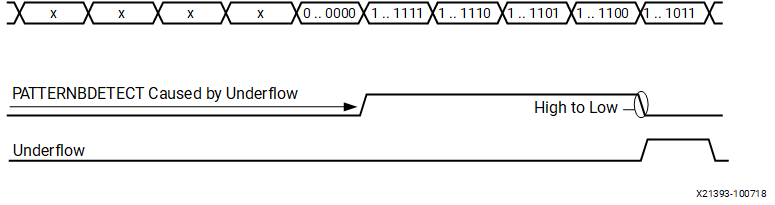# Overflow and Underflow Logic

## Versal ACAP DSP Engine Architecture Manual (AM004)

Document ID
AM004
Release Date
2022-09-11
Revision
1.2.1 English

The following discussion of overflow and underflow applies to sequential accumulators (MACC or Adder-Accumulator) implemented in a single DSP58. The accumulator must have at least one guard bit. When the pattern detector is set to detect a pattern equal to `00000…0` with a mask of `0011111 …1` (default settings), DSP58 flags overflow beyond `00111 … 1` or underflow beyond `11000… 0`. The USE_PATTERN_DETECT attribute is set to PATDET to enable the use of the pattern detect logic. This overflow/underflow implementation uses a redundant sign bit and reduces the output bit width to 57 bits.

Figure 1. Overflow/Underflow Logic in Pattern DetectBy setting the mask to other values like `0000111 …1`, the bit value P[N] at which overflow is detected can be changed. This logic supports saturation to a positive number of 2N –1 and a negative number of 2N in two’s complement where N is the number of 1s in the mask field.

To check overflow/underflow condition for N = 2, the following example is used:

• Mask is set to `0...11`.
• The (N) LSB bits are not considered for the comparison.
• For N = 2, the legal values (patterns) are 22–1 to –22, or 3 to –4.

See the following figures for overflow and underflow examples. The pattern detect logic asserts the overflow/underflow signal for only one clock cycle in the same cycle in which the P output that caused the overflow/underflow is produced.

Figure 2. Overflow Condition in the Pattern DetectorFigure 3. Underflow Condition in the Pattern Detector• PATTERNDETECT is `1` if P = = pattern or mask
• PATTERNBDETECT is a `1` if P = = patternb or mask

Overflow is caused by addition when the value at the output of the adder/subtracter/logic unit goes over 3. Adding 1 to the final value of `0..0011` gives `0..0100` as the result, which causes the PATTERNDETECT output to go to 0. When the PATTERNDETECT output goes from `1` to `0`, an overflow is flagged.

Underflow is caused by subtraction when the value goes below –4. Subtracting 1 from `1..1100` yields `1..1011` (–5), which causes the PATTERNBDETECT output to go to `0`. When the PATTERNBDETECT output goes from `1` to `0`, an underflow is flagged.